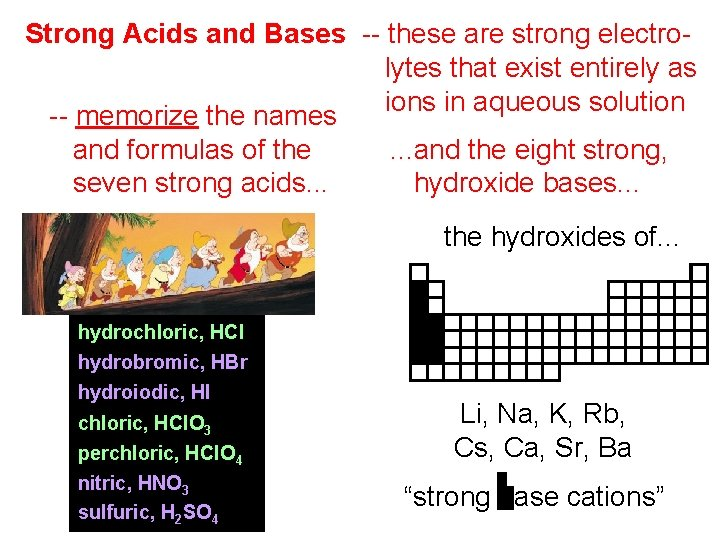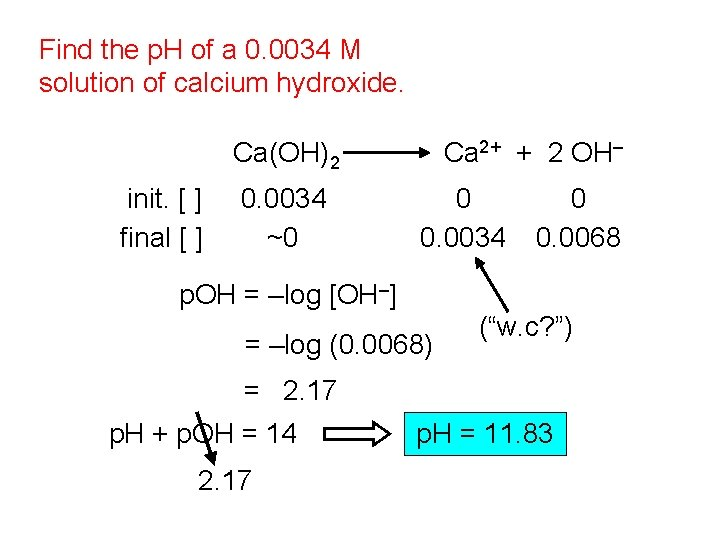# Strong Acids and Bases these are strong electrolytes

• Slides: 6Strong Acids and Bases -- these are strong electrolytes that exist entirely as ions in aqueous solution -- memorize the names. . . and the eight strong, and formulas of the hydroxide bases. . . seven strong acids. . . the hydroxides of. . . hydrochloric, HCl hydrobromic, HBr hydroiodic, HI chloric, HCl. O 3 perchloric, HCl. O 4 nitric, HNO 3 sulfuric, H 2 SO 4 Li, Na, K, Rb, Cs, Ca, Sr, Ba “strong base cations”Strong electrolytes are often written using a one-sided arrow. e. g. , HNO 3(aq) H+(aq) + NO 3–(aq) Find the p. H of a 0. 012 M perchloric acid solution. HCl. O 4 init. [ ] final [ ] 0. 012 ~0 H+ + Cl. O 4– 0 0 0. 012 p. H = –log [H+] = –log (0. 012) = 1. 92 (“Who cares? ”) oo o pp pIf a nitric acid solution has p. H = 2. 66, find the solution’s concentration. [H+] = 10–p. H = 10– 2. 66 = 2. 2 x 10– 3 M HNO 3 H+ + NO 3– So orig. [HNO 3] was also 2. 2 x 10– 3 M. Equipment for the production of nitric acid, formerly part of a U. S. military munitions plant in Joliet, IL.** NOTE: Ca(OH)2, Sr(OH)2, and Ba(OH)2 are strong electrolytes, but have limited solubilities. This means that if you put a big chunk of any of these into water, little of the chunk will dissolve, BUT the little that does dissolve will float around as X 2+(aq) and OH–(aq), NOT as X(OH)2(aq). Barium hydroxide is used in the titration of weak acids, particularly organic acids, because a clear solution of Ba(OH)2 – unlike Na. OH or KOH – will NOT contain any carbonates; carbonates would introduce errors into an analytical titration because the CO 32– ion acts as a weak base.Find the p. H of a 0. 0034 M solution of calcium hydroxide. Ca(OH)2 init. [ ] final [ ] 0. 0034 ~0 Ca 2+ + 2 OH– 0 0. 0034 0 0. 0068 p. OH = –log [OH–] = –log (0. 0068) (“w. c? ”) = 2. 17 p. H + p. OH = 14 2. 17 p. H = 11. 83Metal oxides, hydrides, and nitrides (containing O 2–, H–, and N 3–, respectively) also react with water to produce strongly basic solutions. e. g. , -- O 2–(aq) + H 2 O(l) 2 OH–(aq) -- H–(aq) + H 2 O(l) H 2(g) + OH–(aq) -- N 3–(aq) + 3 H 2 O(l) OH! OH! OH! OH! OH! NH 3(aq) + 3 OH–(aq)